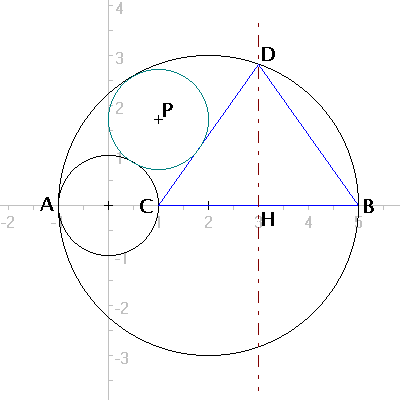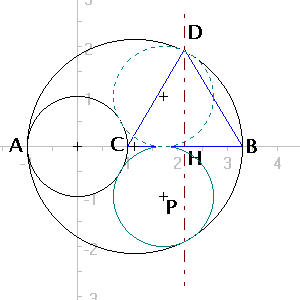## Revisiting a Sangaku Problem

Consider a circle O with a diameter AB. Draw a second circle with diameter AC, such that C is on AB in circle O. Draw an isosceles triangle with base CB and third vertex on circle O. Draw a third circle, tangent to the first three figures. If the radii of the two internal circles are the same, find AC.

Source: Related to past problem.

Solutions were received from Philippe Fondanaiche, Radu Ionescu, Alessandro Fogliati, Jozef Hanenberg, Dan Chirica.

Everyone found that the radii of the small circles is exactly 1/3 of the large circle.  Radu Ionescu' solution builds upon the previous problem:

If we look at Radu Casian Ionescu's solution of "A Sangaku Problem":
" x=(2r(R-r))/(R+r) " take r=x ==> r=R/3 ==> AC=2R/3

Alessandro Fogliati found an additional solution that meets the stated problem requirements.  His two soltutions are here:

 AC = AB/3AC=AB*3/(3+sqrt(10)) [provided I did my math right - KD].Mail to Ken